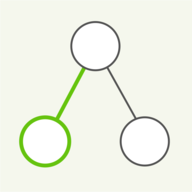7 Jun 2019

# Various sorting algorithms

If you are familiar with my page on Quicksort, you already know one of the leading algorithms for sorting numbers. This page presents some simpler, more intuitive but generally slower algorithms for sorting. They are so simple that they do not need a separate page each, just to explain them, so they have been summarized here all on one page. We will have a look at Bubble sort, Insertion sort, Selection sort, Counting sort and Bucket sort. In each case we are sorting for an ascending order, placing the largest elements at the right end of the sorted array. Alternatively we could have chosen a descending sort, leading to the opposite order.## Algorithm: Bubble sort

Bubble sort is named after the fact that the values to be sorted ‘bubble up’ through the array. We start at the front of the array and compare the first two values. If the first one is larger than the second, swap them. The values being compared are highlighted as green columns above. The larger of the two is then compared to the next value in the array. So we continue to compare and possibly swap values through the array until the largest value ends up at the end. As an optimisation the partially sorted right end of the array is highlighted as green number boxes, and once this part is reached, the bubbling up is over.## Algorithm: Insertion sort

Insertion sort looks very similar to Bubble sort, but is indeed a different algorithm and works differently. Insertion sort has in common with Bubble sort that the left half of the array is unsorted and the right side is already partially sorted. As the right half grows from zero length to covering the entire array, the array ends up sorted. To grow the sorted end, the last element in the unsorted side is repeatedly swapped through the sorted part until it has found its right position. It is so inserted at the right point.## Algorithm: Selection sort

Selection sort is the algorithm closest to what people first think of when asked to sort a list of numbers automatically. It repeatedly selects the largest of the remaining entries and places it as the end of the sorted part of the array, by swapping it to the right position. Selecting the largest element is done simply by traversing the unsorted side once completely. The traversal is indicated by the green column, the currently largest candidate is indicated in blue. As before, the already-sorted part is indicated by the green background in the number boxes.## Algorithm: Counting sort

The sorting methods we have seen so far, are called comparison-based methods. They are based on comparing entries on our array and swapping their order based on the result of such comparisons. The counting sort algorithm is different. The sorting is based on the absolute value of the entries. It works by counting out the number of occurances of each value and then determining the right position in the array from the counts. This works best when the number of distinct values is not too large, so for this demonstration we have limited the range of values to integers between 1 and 31. The panel above shows how the input array is traversed once to compute the counts. To see the entire sorting algorithm, have a look at the next panel.## Using counts to sort

The second phase of Counting sort moves through the counts and places the appropriate number of elements into the now empty array, which we are sorting. Due to the correspondence between the counts (below) and the main array (above) the end result is the sorted array. This method seems fast, and indeed it is. But we had to make some restrictions for it to work this well, such as using a limited number of random values in the input array. We will introduce one more sorting method below, Bucket sort, which can do away with this restriction, but otherwise works similar to Counting sort.## Algorithm: Bucket sort

Bucket sort places each of the array elements into a bucket. The right bucket position is calculated from the value in question, the number of buckets and the expected value range. How many buckets need to be used is a design factor of the algorithm. The main assumption used by bucket sort, is that the values are distributed more or less evenly, so that they don't all end up in the same few buckets. Once the array is split up like so, each bucket needs to be sorted individually. This can be done in parallel and can be fairly quick as there are not many values per bucket. The final step is to concatenate all the values from the buckets back into the array. Et voilà!

If you enjoyed this page, there are more algorithms and data structures to be found on the main page. And if you have still not had enough of sorting, the page about the Heap includes a panel on Heapsort, yet another sorting algorithm.